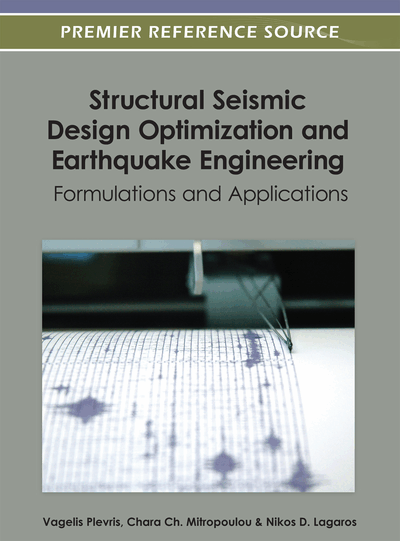# Fuzzy Identification of Seismically Excited Smart Systems

JinSeop Kim (Massachusetts Institute of Technology, USA), Yeesock Kim (Worcester Polytechnic Institute, USA) and Tahar El-Korchi (Worcester Polytechnic Institute, USA)
DOI: 10.4018/978-1-4666-1640-0.ch013
Available
\$33.75
List Price: \$37.50
10% Discount:-\$3.75
TOTAL SAVINGS: \$3.75

## Abstract

In this chapter, a nonlinear modeling framework to identify nonlinear behavior of smart structural systems under seismic excitations is proposed. To this end, multi-input-multi-output (MIMO) autoregressive exogenous (ARX) input models and Takagi-Sugeno (TS) fuzzy models are coalesced as the MIMO ARX-TS fuzzy model. The premised part of the proposed MIMO ARX-TS fuzzy model is optimized using the hierarchical clustering (HRC) algorithm, while its consequent parameters are optimized via the weighted linear least squares estimation. The performance of the proposed model is investigated using the dynamic response of a three-story shear planer frame structure equipped with a magnetorheological (MR) damper subject to earthquake disturbances. Furthermore, the impact of the HRC algorithm on the performance of the MIMO ARX-TS fuzzy model is compared with that of the subtractive and the fuzzy C-means clustering algorithms. The equivalence of the original and identified data is numerically shown to prove that the HRC MIMO ARX-TS fuzzy model introduced here is effective in estimating nonlinear behavior of a seismically excited building-MR damper system.
Chapter Preview
Top

## 1. Introduction

The development of an accurate explicit mathematical model of dynamical systems is one of the most important tasks in structural health monitoring and control system design for hazard prediction and mitigation of dynamical systems because precise mathematical information related to the dynamic systems is used for damage prediction and/or calculation of control forces (Kerber et al. 2007; Yen & Langari 1998; Lin et al. 2001; Bani-Hani 1999; Kim et al. 2011). However, it is still challenging to derive a mathematical model of nonlinear dynamic systems (Moon & Aktan 2006). An example of such nonlinear dynamic systems can be sought when highly nonlinear hysteretic actuators/dampers are applied to structural systems for efficient energy dissipation: the structure integrated with the nonlinear control devices behaves nonlinearly although the structure itself is usually assumed to remain linear (Yi et al. 2001; Ramallo et al. 2004). Because the structure integrated with the nonlinear hysteretic control device is intrinsically nonlinear, it is challenging to develop an appropriate mathematical model for the integrated nonlinear system including the interaction effects between the structural system and the nonlinear control device while it plays a key role in both structural health monitoring and controlling system (Dyke et al. 1998).

## Complete Chapter List

Search this Book:
Reset